# 如何构建一棵红黑树(分析TreeMap源码)

## 二叉树

class Entry<K, V> {
K key;
V value;
Entry left;
Entry right;
}


## 二叉搜索树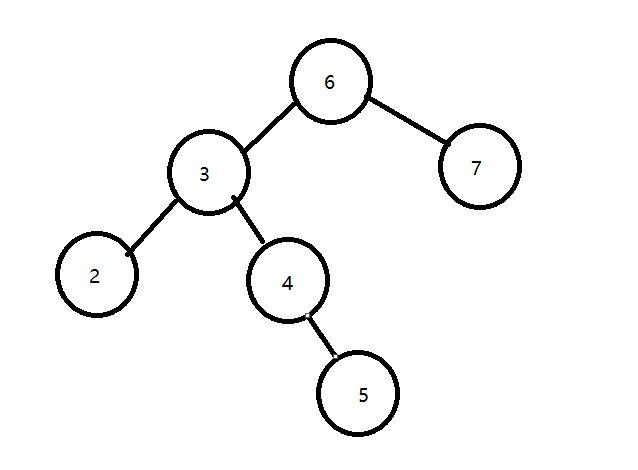## 红黑树

### 五个性质

• 节点是红色或黑色。
• 根节点是黑色。
• 所有叶子都是黑色。（叶子是NUIL节点）。
• 每个红色节点的两个子节点都是黑色。（从每个叶子到根的所有路径上不能有两个连续的红色节点）。
• 从任一节点到其每个叶子的所有路径都包含相同数目的黑色节点。

• 规定路径上不能有两个连续的红色节点。这样最短的可能路径都是黑色节点，最长的可能路径有交替的红色和黑色节点。
• 所有最长的路径都有相同数目的黑色节点，这就使得没有路径能多于任何其他路径的两倍。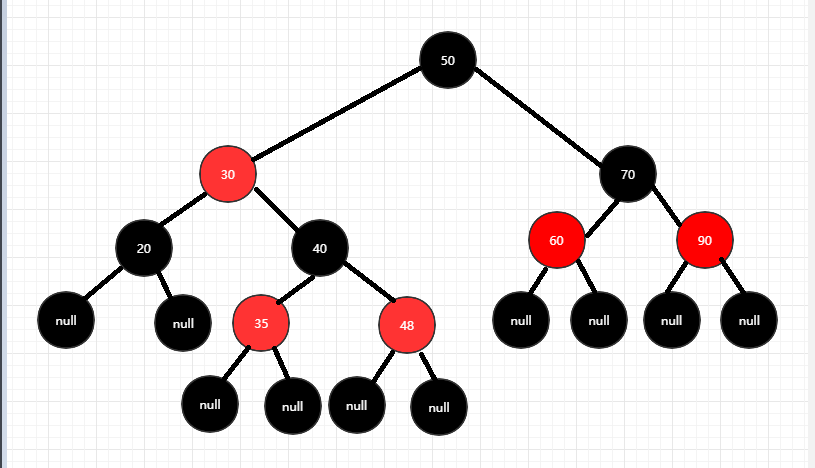### 红黑树的插入

#### 染色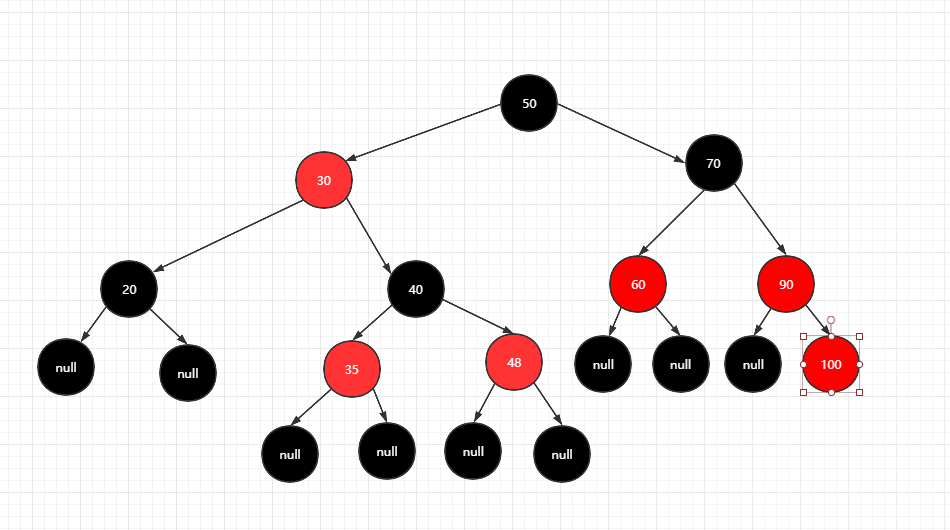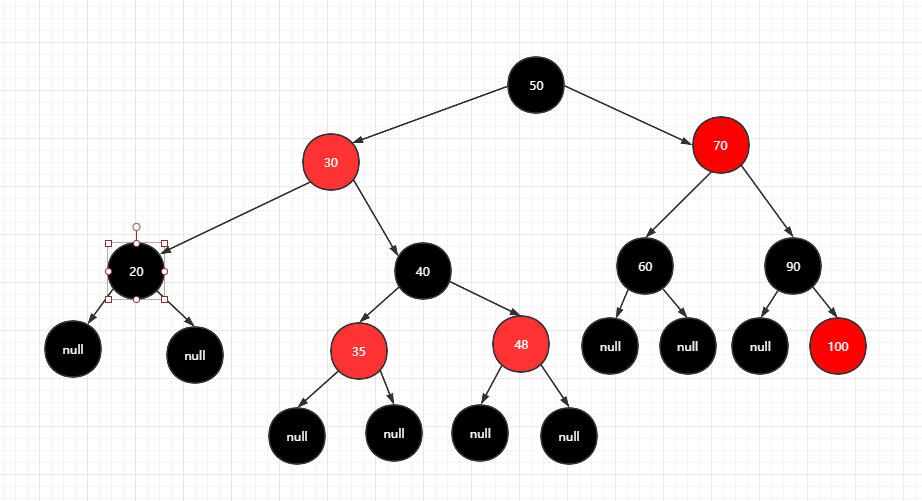#### 旋转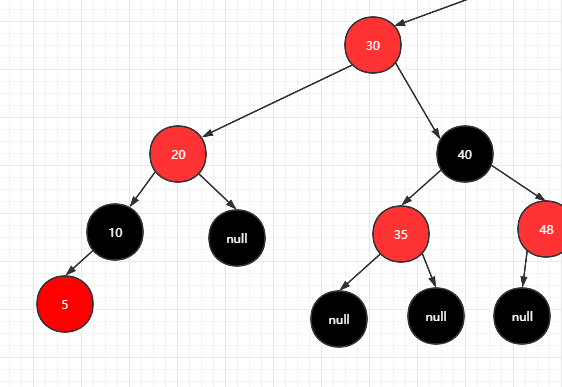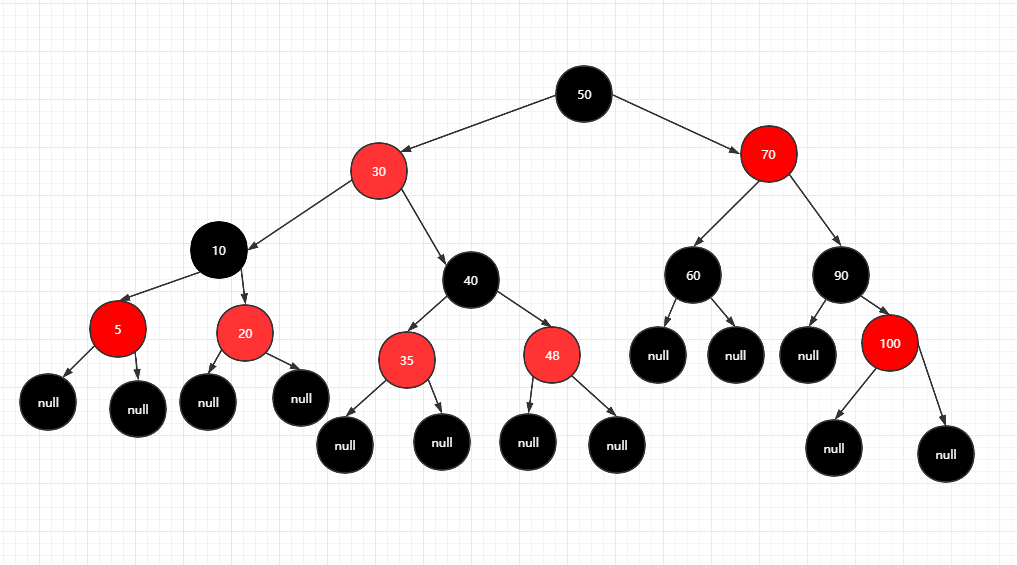##### 左转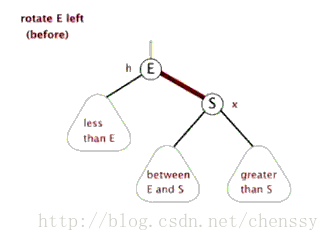##### 右转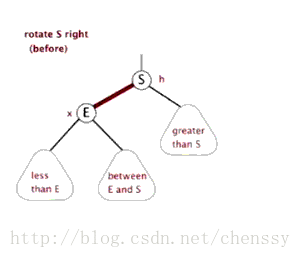## 代码实现

TreeMap基于红黑树（Red-Black tree）实现。该映射根据其键的自然顺序进行排序，或者根据创建映射时提供的
Comparator 进行排序，具体取决于使用的构造方法。

public class tree<K, V> {
}


### 构建树

##### 变量
//根节点
private transient Entry<K, V> root;
//定义节点黑色
private static final boolean BLACK = true;
//定义节点红色
private static final boolean RED = false;
//map的长度
private static int size;


private final Comparator<? super K> comparator;


//如果外部实现了Comparator类，采用传入的Comparator进行排序
public tree(Comparator<? super K> comparator) {
this.comparator = comparator;
}
//默认Comparator为null
public tree() {
this.comparator = null;
}

##### 定义Entry
tatic final class Entry<K, V> {
//key
private K key;
//值
private V value;
//左子树
private Entry left;
//右子树
private Entry right;
//父节点
private Entry parent;
//默认树的节点都是黑,即null为黑
boolean color = BLACK;
// 构造方法
public Entry() {
}
public Entry(K key, V value, Entry parent) {
this.key = key;
this.value = value;
this.parent = parent;
}

}


 public V setValue(V value) {
V oldv = this.value;
this.value = value;
return oldv;
}

##### 定义put方法

 public V put(K key, V value) {
}

###### root节点

   Entry<K, V> r = root;
//根节点
if (root == null) {
//判断是否为空
compare(key, key);
root = new Entry<>(key, value, null);
size = 1;
return null;
}


compare方法即能满足对空值的判断，也能对两个key进行判断，这个方法是我源码最喜欢的方法之一，以后编码的时候借鉴

private int compare(Object k1, Object k2) {
//这里要求了key是实现了Comparable接口，如果有实现那就调用实现的对比，如果没有那就使用key的实现的compareTo对比方法
return comparator == null ?
((Comparable<? super K>) k1).compareTo((K) k2) :
comparator.compare((K) k1, (K) k2);
}

###### 寻找parent节点
  Entry<K, V> parent;
int cmp;
Comparator<? super K> compare = comparator;
if (compare != null) {
do {
parent = r;
cmp = compare.compare(key, r.key);
if (cmp < 0) {
r = r.left;
} else if (cmp > 0) {
r = r.right;
} else
return r.setValue(value);
} while (r != null);
} else {
if (key == null) {
throw new NullPointerException();
}
Comparable<? super K> k = (Comparable<? super K>) key;
do {
parent = r;
cmp = k.compareTo(r.key);
if (cmp < 0) {
r = r.left;
} else if (cmp > 0) {
r = r.right;
} else
return r.setValue(value);
} while (r != null);
}


 Entry<K, V> parent;
int cmp;
......
do {
parent = r;
cmp = k.compareTo(r.key);
if (cmp < 0) {
//小于0在左边
r = r.left;
} else if (cmp > 0) {
//对于0在右边
r = r.right;
} else
return r.setValue(value);
} while (r != null);

###### 插入

 Entry<K, V> e = new Entry<>(key, value, parent);
if (cmp < 0) {
parent.left = e;
} else {
parent.right = e;
}
size++;


### 调整

private void fixAfterInsertion(Entry<K, V> x) {
x.color = RED;
//调整方法
{
}
root.color =  BLACK;
}


###### 调整条件
while (x != null && x != root && x.parent.color == RED) {
}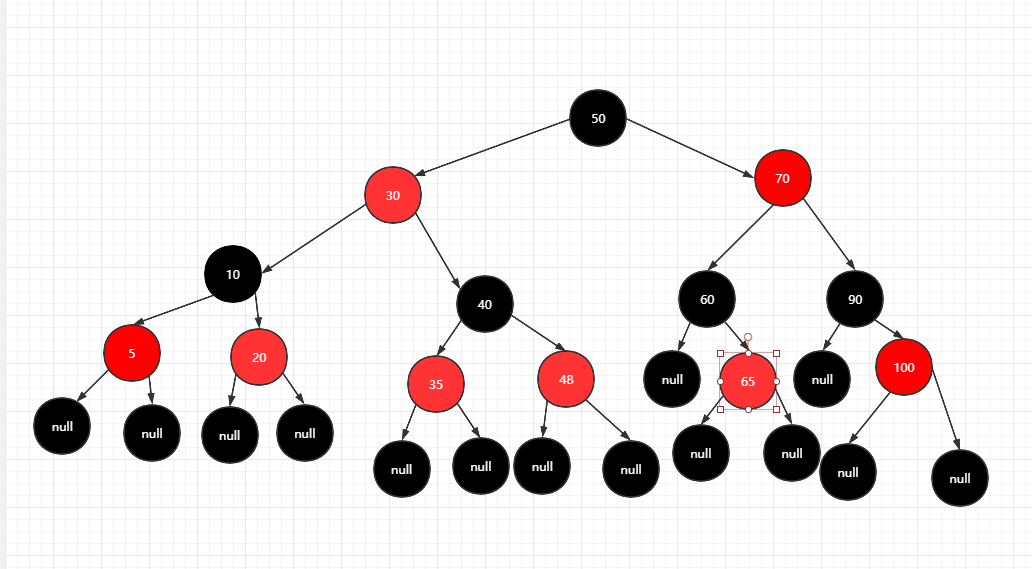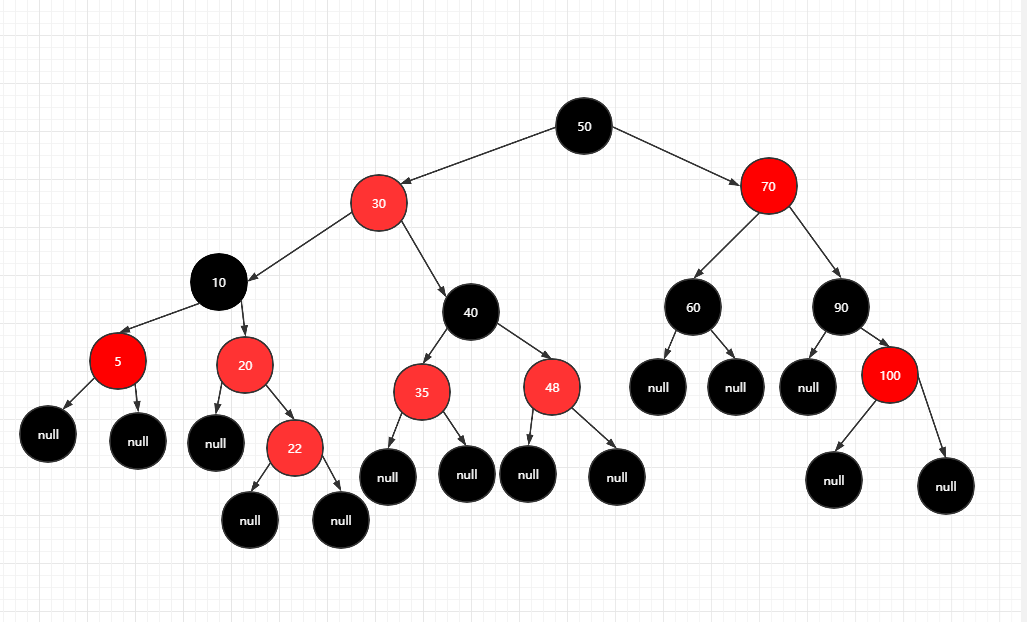###### 染色操作

1.判断当前父节点，是在爷节点(父节点的父节点)的左边还是右边，这里会涉及到后面是左旋还是右旋，染色操作和当前节点是否左节点还是右节点无关联。

if (parentOf(x) == leftOf(parentOf(parentOf(x)))) {}


//返回节点右边节点
private static <K, V> Entry<K, V> rightOf(Entry<K, V> x) {
return x == null ? null : x.left;
}
//返回节点的左节点
private static <K, V> Entry<K, V> leftOf(Entry<K, V> x) {
return x == null ? null : x.left;
}
//返回节点父节点
private static <K, V> Entry<K, V> parentOf(Entry<K, V> x) {
return x == null ? null : x.parent;
}
//设置颜色
private <K, V> void setColor(Entry<K, V> x, boolean color) {
if (x != null) {
x.color = color;
}
}
//获取颜色 默认空节点返回黑色节点，符合第四特性
private <K, V> boolean colorOf(Entry<K, V> x) {
return (x == null ? BLACK : x.color);
}


Entry<K, V> y = rightOf(parentOf(parentOf(x)));
if (colorOf(y) == RED) {
setColor(parentOf(x),BLACK);
setColor(y,BLACK);
setColor(parentOf(parentOf(x)),RED);
x=parentOf(parentOf(x));
}

Entry<K, V> y = leftOf(parentOf(parentOf(x)));
if (colorOf(y)  == RED) {
setColor(parentOf(x),BLACK);
setColor(y,BLACK);
setColor(parentOf(parentOf(x)),RED);
x=parentOf(parentOf(x));
}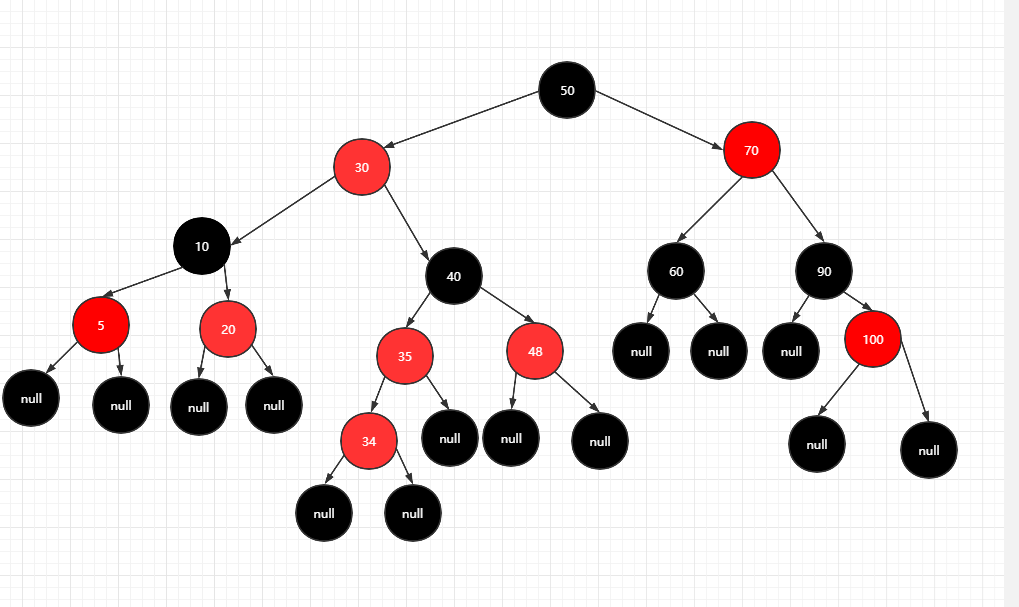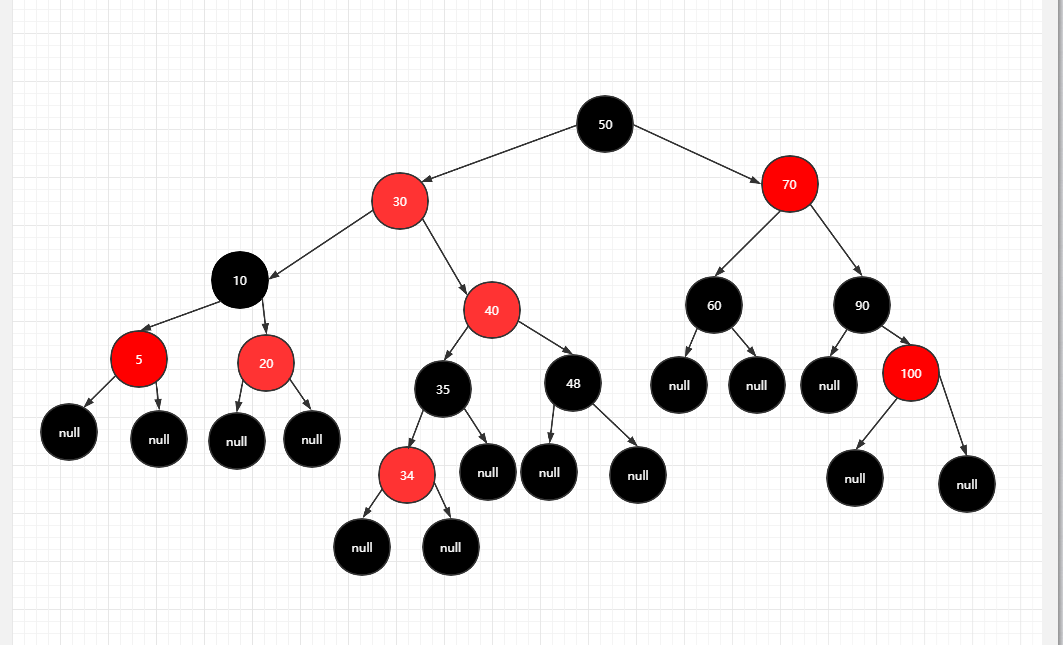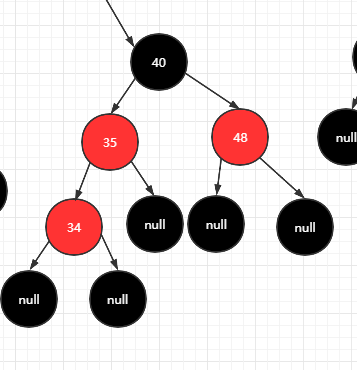1. 34和35节点必须有个节点变黑，破坏第四特性。
2. 假设35变黑，左边增加了一个黑色节点，破坏了40节点的第五特性，这时我们在右边也增加一个黑色节点(48变黑)，保证了40节点的第五特性，
3. 但是破坏了40父节点的第五特性，每个路径增加一个黑色节点，
4. 这个时候，我们就要想办法保证40父节点的第五特性，也要保证40节点的平衡，那就去掉一个路径上共同节点40。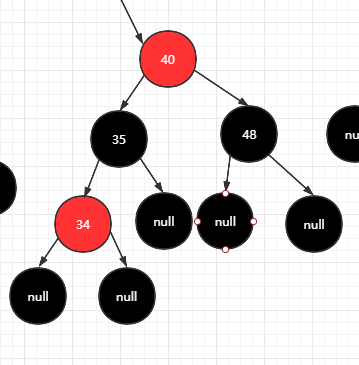1. 将父节点和叔节点染黑
2. 将爷节点染红
3. 将循环的节点变为爷节点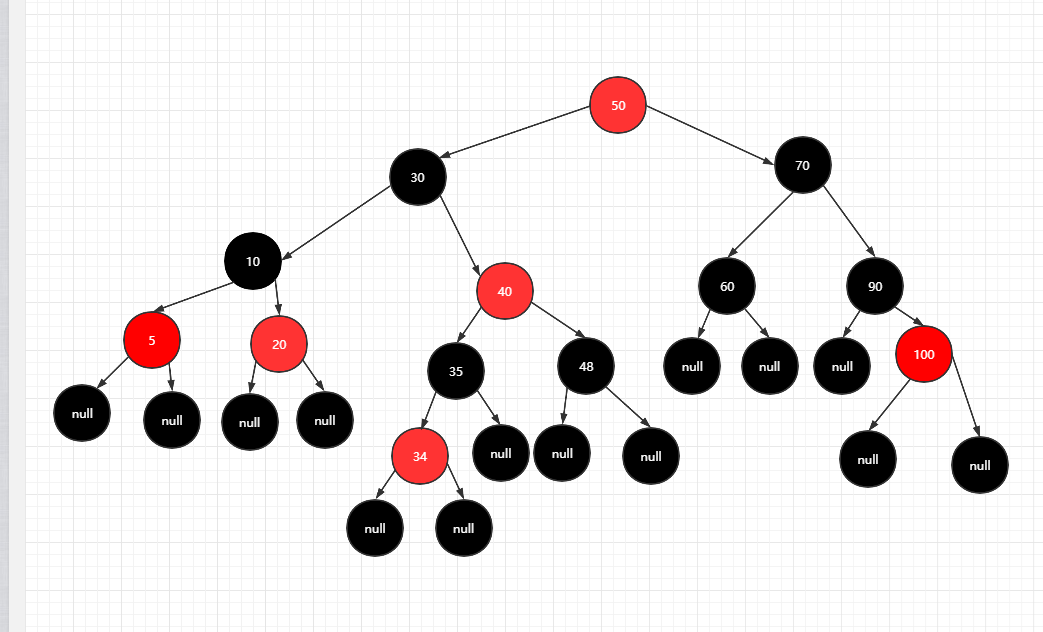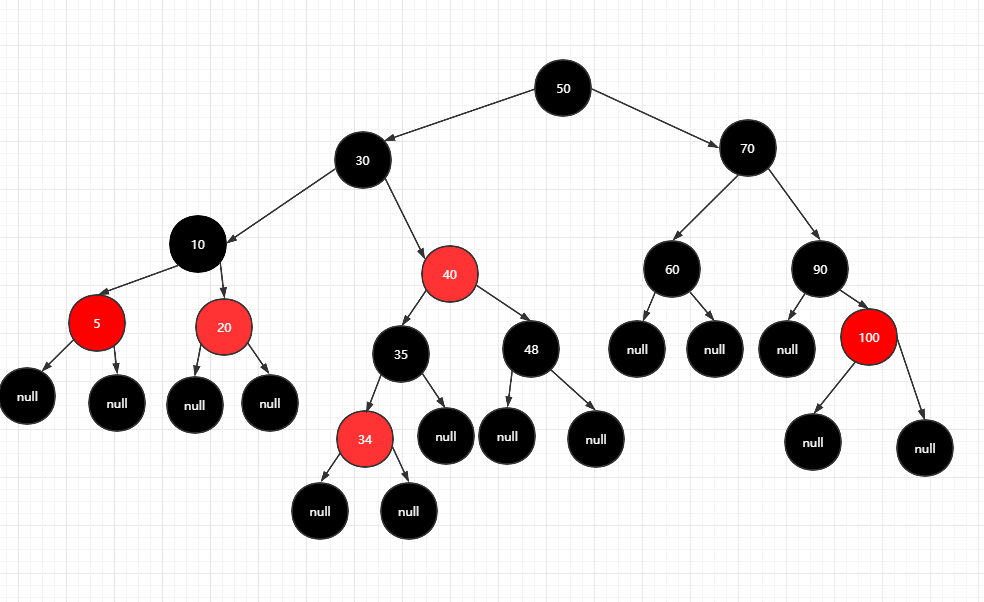###### 旋转操作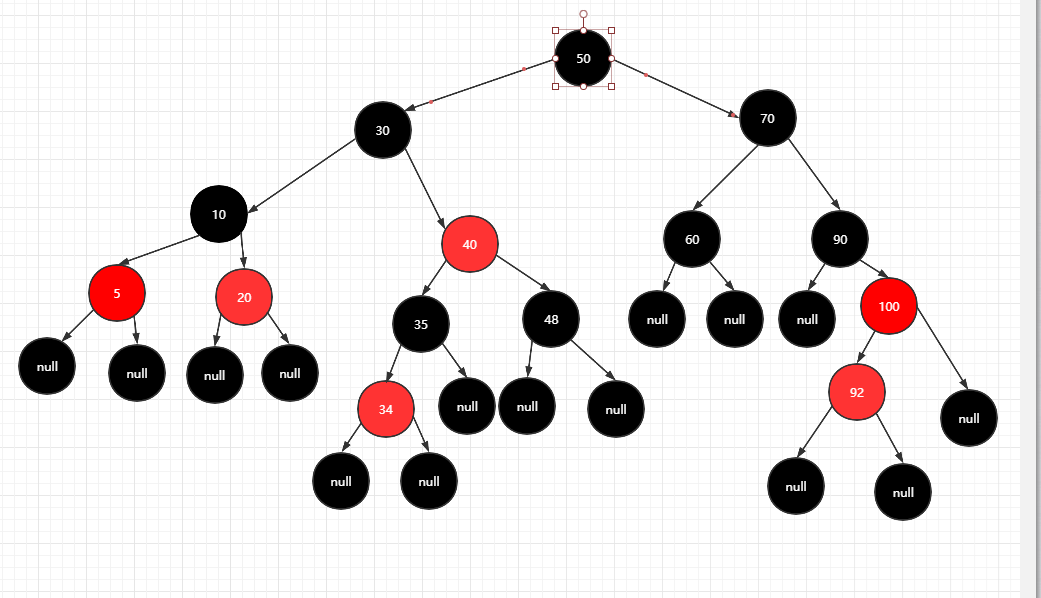1. 92节点只能右旋，需要满足二叉搜索树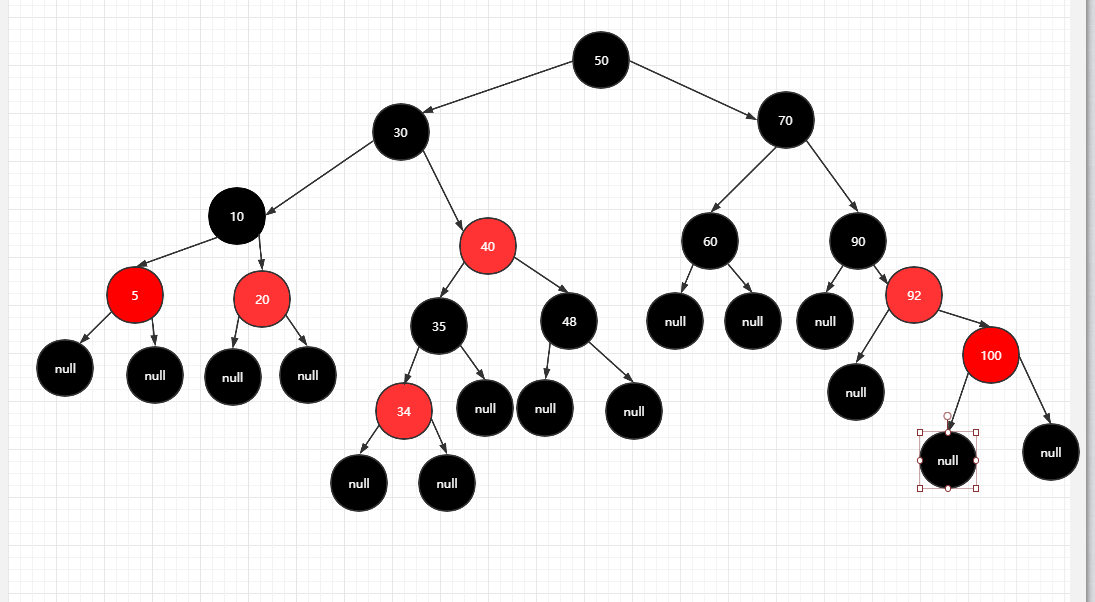2. 92节点再左旋，使得90成为其左子树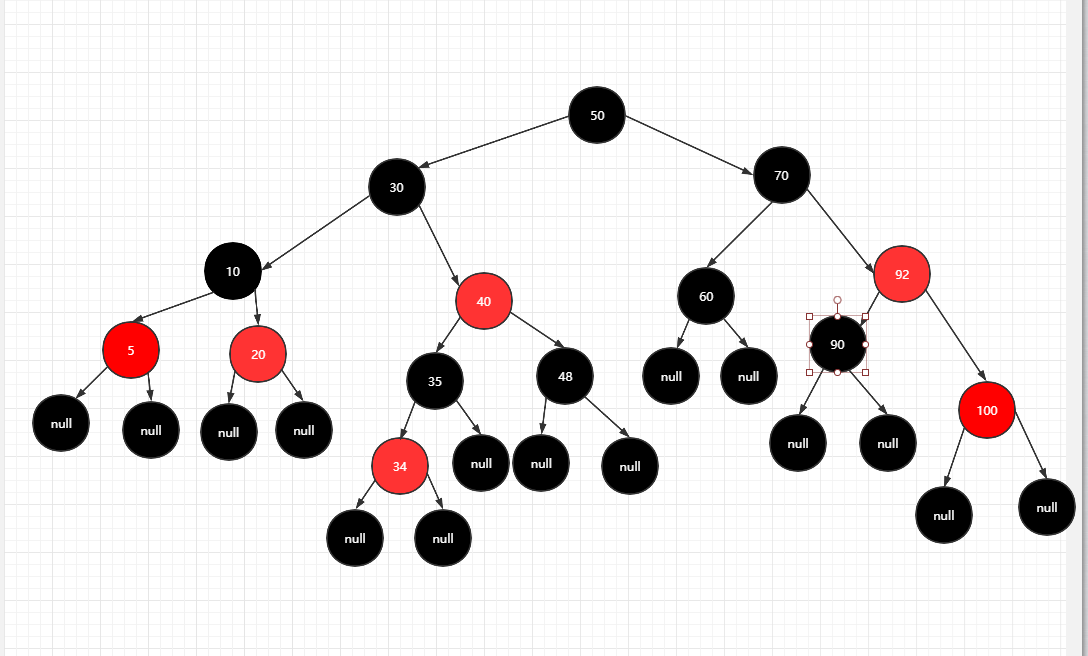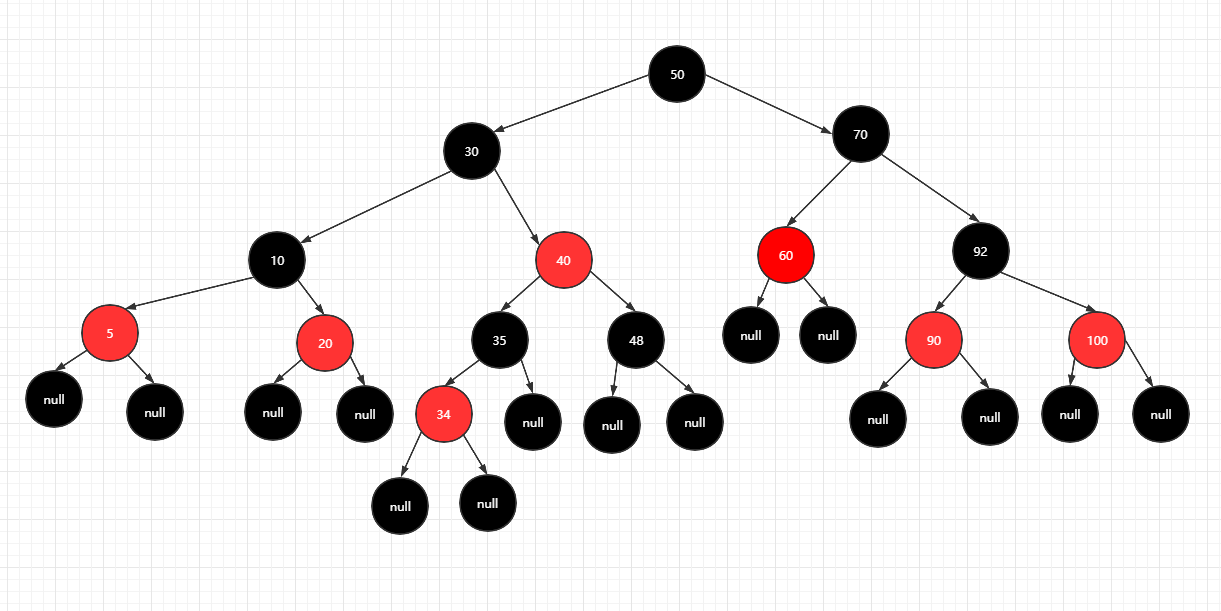if (parentOf(x) == leftOf(parentOf(parentOf(x)))) {
......
}
else{
Entry<K, V> y = leftOf(parentOf(parentOf(x)));
if (colorOf(y)  == RED) {
......
}else{
//当前节点是父节点的左子树，需要右旋
if(x==leftOf(parentOf(x))){
x=parentOf(x);
rotateRight(x);
}
//此时x节点为旋转前父节点，通过右旋，父节点在插入节点的右字子树上,参考下图
setColor(parentOf(x),BLACK);
setColor(parentOf(parentOf(x)),RED);
rotateLeft(parentOf(parentOf(x)));
}
}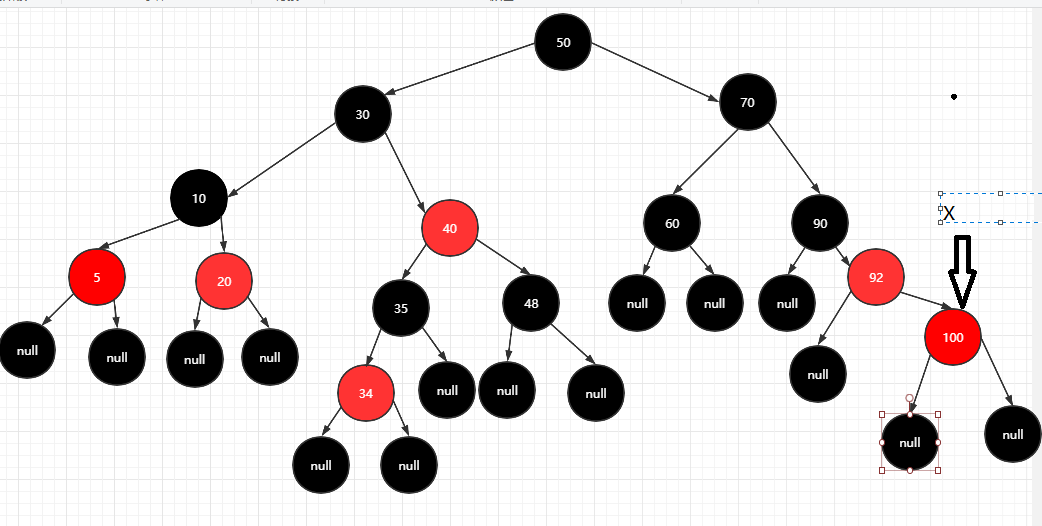private void rotateRight(Entry<K,V> p) {
if (p != null) {
Entry<K, V> l = p.left;
p.left = l.right;
if (l.right != null) {
//更换父节点
l.right.parent = p;
}
//互换父节点
l.parent = p.parent;
if (p.parent == null) {
root = l;

} else if (p == leftOf(parentOf(p))) {
parentOf(p).left = l;
} else {
parentOf(p).right = l;
}
//p的节点换成l
p.parent = l;
//l的左边节点换成p
l.right = p;
}
}private void rotateLeft(Entry<K,V> p) {
if (p != null) {
Entry<K, V> r = p.right;
p.right = r.left;
if (r.left != null) {
//更换父节点
r.left.parent = p;
}
//互换父节点
r.parent = p.parent;
if (p.parent == null) {
root = r;
} else if (p == leftOf(parentOf(p))) {
parentOf(p).left = r;
} else {
parentOf(p).right = r;
}
//p的节点换成l
p.parent = r;
//l的左边节点换成p
r.left = p;
}
}

###### 为什么要旋转？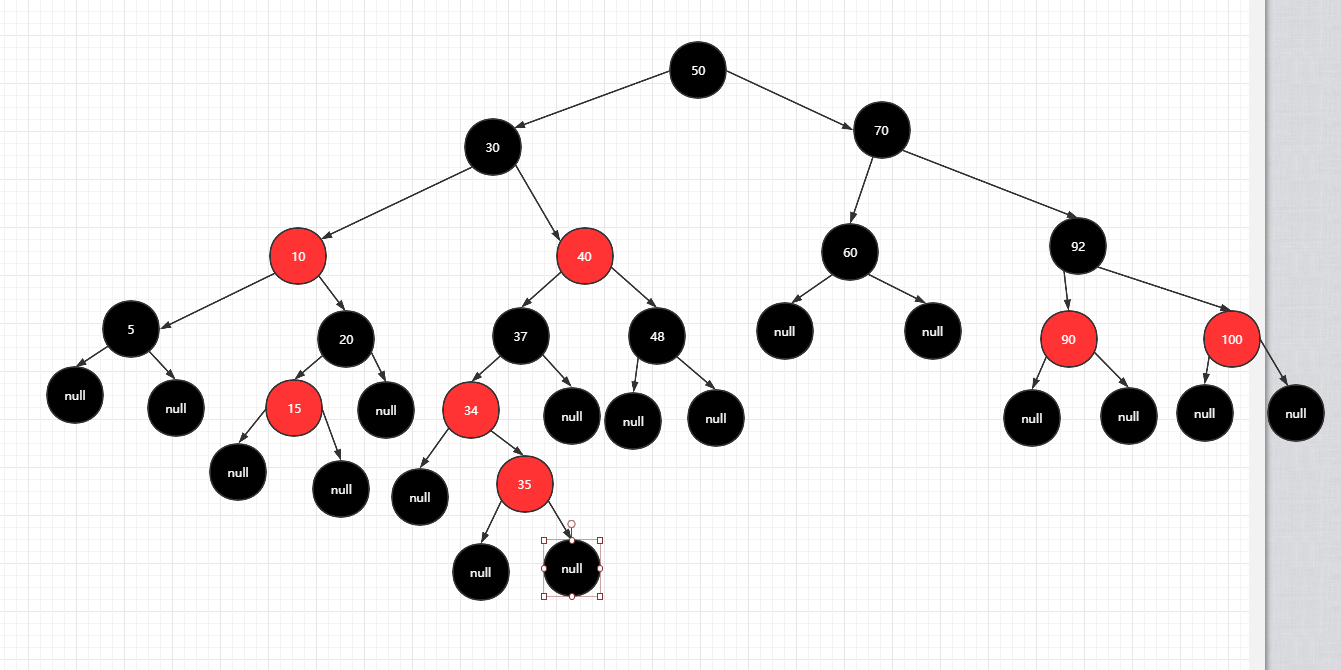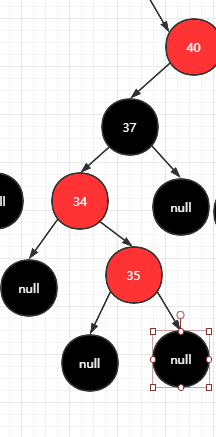35节点和34节点肯定有一个节点需要变黑，才能不违背第四特性(每个红色节点的两个子节点都是黑色)；

1. 37变红，减少两边节点的黑色个数，保证平衡。*违背第四特性，这思路pass
2. 那就减少37右子树的黑色节点个数，但是37右子树是null,参考红黑树第五特性，插入节点的位置的叔节点大部分情况都是null,这个思路也pass.
什么样子的红黑树才是最适合变色的？
参照染色的规则，叔节点和父亲节点都是红色，那就思考为什么这个结构是可以染色解决问题？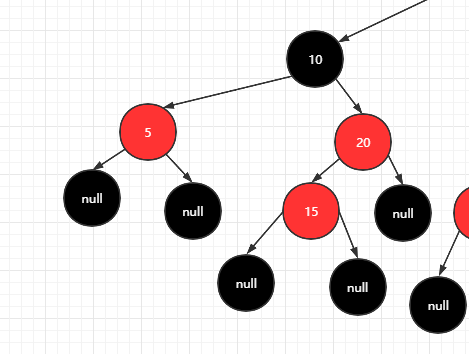1.增加5节点为黑色，10节点的左右路径上各增加黑色
2.需要减少一个公共的节点黑色，保证左右路径平衡，10变红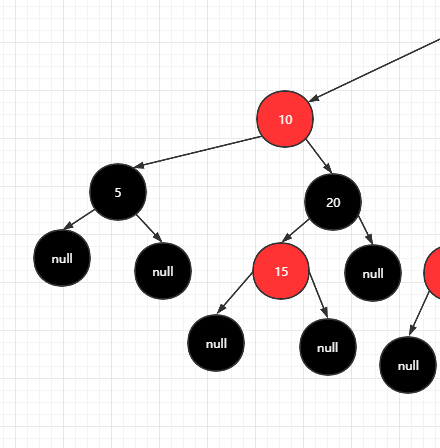1.只在在影响平衡的节点上进行调整，一般是三个
2.调整不平衡节点为一个二叉搜索树，并且左右有一个节点是红色，方便染色操作

1.找出影响的节点，37-34-35
2.调整不平衡节点为一个二叉搜索树

 35
/  \
34  37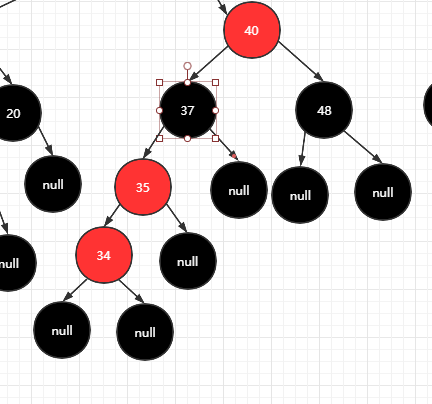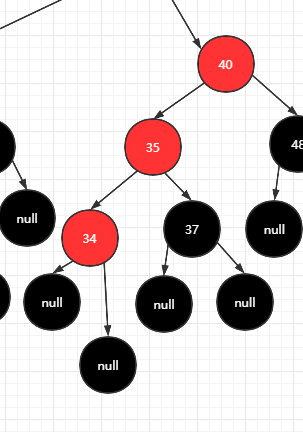1.35和34必须有一个节点变黑，保证第四特性
2.只能35节点变黑，左子树无影响，但是其右路径37是黑色，影响了平衡，将37染红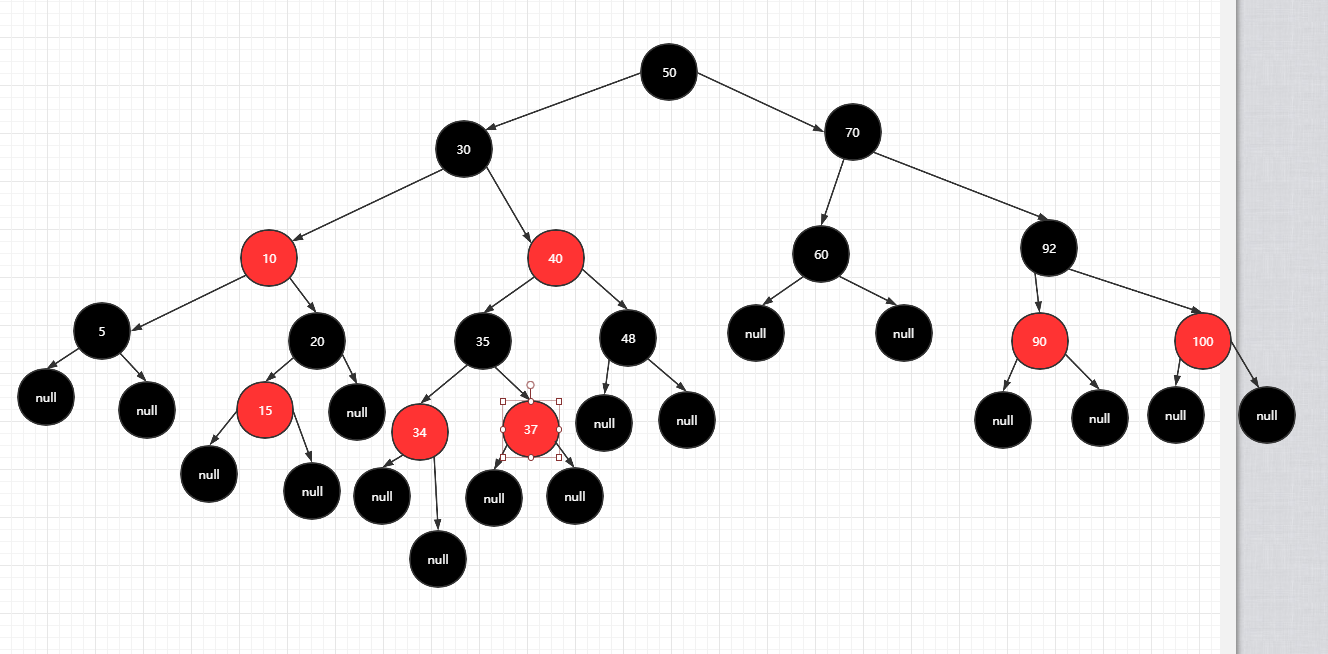//如果当前节点在父节点的右边，大于父节点，在构建二叉树的取一个中间值作为父节点
if(x==rightOf(parentOf(x))){
x=parentOf(x);
rotateLeft(x);
}


parentOf(x)x<parentOf(parentOf(x)；

parentOf(parentOf(x))
/
parentOf(x)
\
x


setColor(parentOf(x),BLACK);
setColor(parentOf(parentOf(x)),RED);
rotateRight(parentOf(parentOf(x)));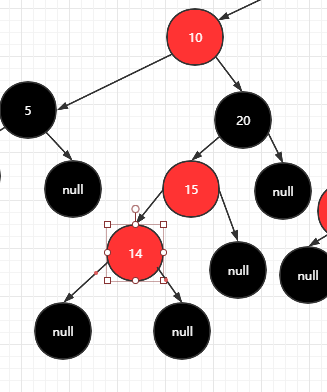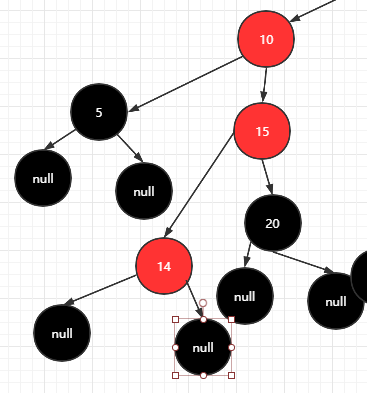14和15节点肯定要变黑一个，14和20肯定是15的左右子树；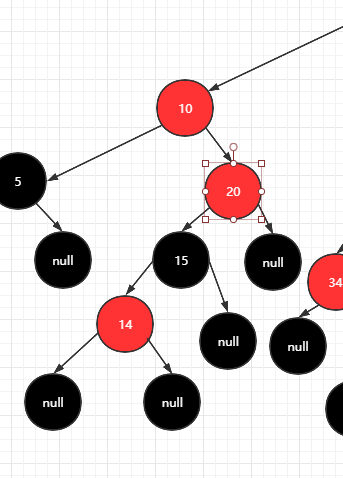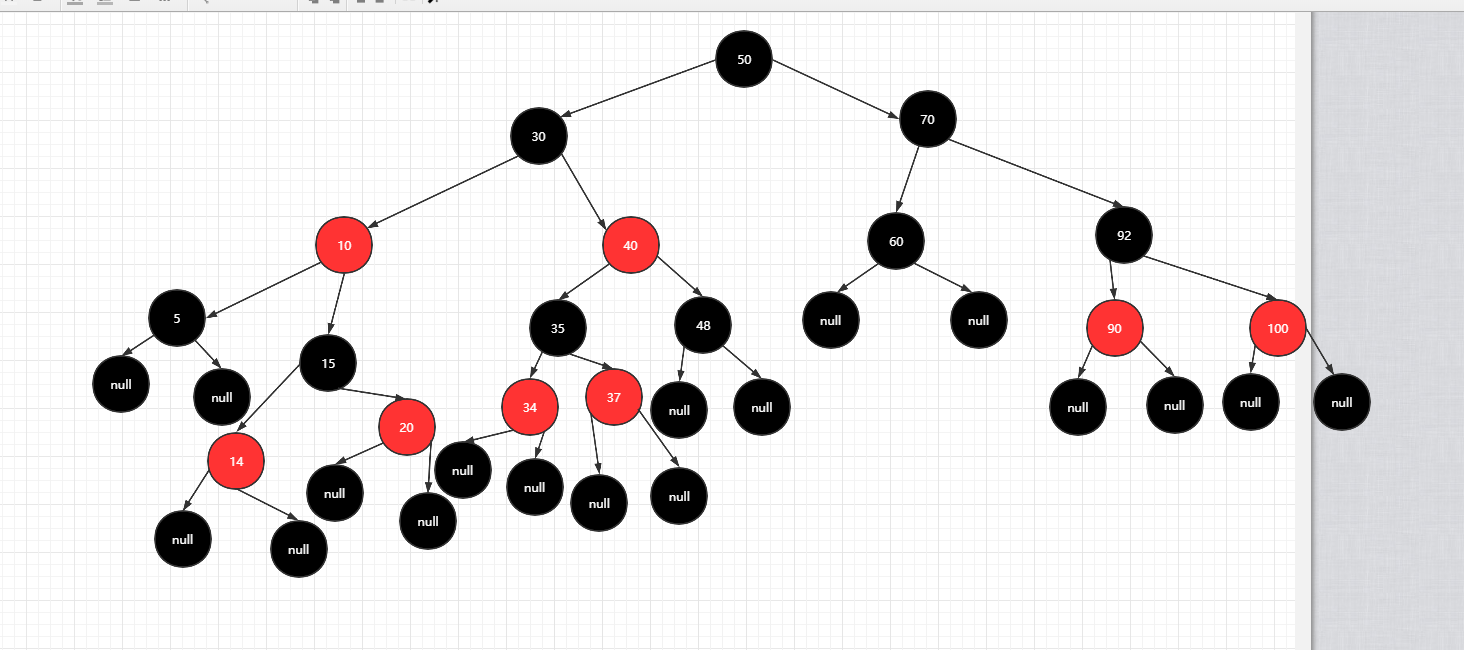### 源码

public class tree<K, V> {

private static final boolean BLACK = true;

private static final boolean RED = false;

private static int size;

/**
* 根节点
*/
private transient Entry<K, V> root;

//对比

private final Comparator<? super K> comparator;

/**
* 构造方法
*
* @param comparator
*/
public tree(Comparator<? super K> comparator) {
this.comparator = comparator;
}

public tree() {
this.comparator = null;
}

public V put(K key, V value) {
Entry<K, V> r = root;
//根节点
if (root == null) {
//判断是否为空
compare(key, key);
root = new Entry<>(key, value, null);
size = 1;
return null;
}
Entry<K, V> parent;
int cmp;
Comparator<? super K> compare = comparator;
if (compare != null) {
do {
parent = r;
cmp = compare.compare(key, r.key);
if (cmp < 0) {
r = r.left;
} else if (cmp > 0) {
r = r.right;
} else
return r.setValue(value);
} while (r != null);
} else {
if (key == null) {
throw new NullPointerException();
}
Comparable<? super K> k = (Comparable<? super K>) key;
do {
parent = r;
cmp = k.compareTo(r.key);
if (cmp < 0) {
r = r.left;
} else if (cmp > 0) {
r = r.right;
} else
return r.setValue(value);
} while (r != null);
}
Entry<K, V> e = new Entry<>(key, value, parent);
if (cmp < 0) {
parent.left = e;
} else {
parent.right = e;
}
size++;
//调整树
fixAfterInsertion(e);
return null;
}

//调整树结构为红黑树
private void fixAfterInsertion(Entry<K, V> x) {
//默认插入的节点都是红色
x.color = RED;
while (x != null && x != root && x.parent.color == RED) {
//判断当前节点是父亲节点的左节点
if (parentOf(x) == leftOf(parentOf(parentOf(x)))) {
Entry<K, V> y = rightOf(parentOf(parentOf(x)));
if (colorOf(y) == RED) {
setColor(parentOf(x),BLACK);
setColor(y,BLACK);
setColor(parentOf(parentOf(x)),RED);
x=parentOf(parentOf(x));
}else {
if(x==rightOf(parentOf(x))){
x=parentOf(x);
rotateLeft(x);
}
setColor(parentOf(x),BLACK);
setColor(parentOf(parentOf(x)),RED);
rotateRight(parentOf(parentOf(x)));
}
}else{
Entry<K, V> y = leftOf(parentOf(parentOf(x)));
if (colorOf(y)  == RED) {
setColor(parentOf(x),BLACK);
setColor(y,BLACK);
setColor(parentOf(parentOf(x)),RED);
x=parentOf(parentOf(x));
}else{
if(x==leftOf(parentOf(x))){
x=parentOf(x);
rotateRight(x);
}
setColor(parentOf(x),BLACK);
setColor(parentOf(parentOf(x)),RED);
rotateLeft(parentOf(parentOf(x)));
}
}
}
root.color =  BLACK;
}
//左旋
private void rotateLeft(Entry<K,V> p) {
if (p != null) {
Entry<K, V> r = p.right;
p.right = r.left;
if (r.left != null) {
//更换父节点
r.left.parent = p;
}
//互换父节点
r.parent = p.parent;
if (p.parent == null) {
root = r;
} else if (p == leftOf(parentOf(p))) {
parentOf(p).left = r;
} else {
parentOf(p).right = r;
}
//p的节点换成l
p.parent = r;
//l的左边节点换成p
r.left = p;
}
}
//右旋
private void rotateRight(Entry<K,V> p) {
if (p != null) {
Entry<K, V> l = p.left;
p.left = l.right;
if (l.right != null) {
//更换父节点
l.right.parent = p;
}
//互换父节点
l.parent = p.parent;
if (p.parent == null) {
root = l;
} else if (p == leftOf(parentOf(p))) {
parentOf(p).left = l;
} else {
parentOf(p).right = l;
}
//p的节点换成l
p.parent = l;
//l的左边节点换成p
l.right = p;
}
}

private <K, V> void setColor(Entry<K, V> x, boolean color) {
if (x != null) {
x.color = color;
}
}

private <K, V> boolean colorOf(Entry<K, V> x) {
return (x == null ? BLACK : x.color);
}

private static <K, V> Entry<K, V> rightOf(Entry<K, V> x) {
return x == null ? null : x.left;
}

private static <K, V> Entry<K, V> leftOf(Entry<K, V> x) {
return x == null ? null : x.left;
}

private static <K, V> Entry<K, V> parentOf(Entry<K, V> x) {
return x == null ? null : x.parent;
}

private int compare(Object k1, Object k2) {
return comparator == null ? ((Comparable<? super K>) k1).compareTo((K) k2) :
comparator.compare((K) k1, (K) k2);
}

/**
* 1.变量
* 2.构造树
* 3.调整树
*/
//树的对象
static final class Entry<K, V> {
private K key;
private V value;
private Entry left;
private Entry right;
private Entry parent;
//默认树的节点都是黑,即null为黑
boolean color = BLACK;

public Entry() {
}

public Entry(K key, V value, Entry parent) {
this.key = key;
this.value = value;
this.parent = parent;
}

public K getKey() {
return key;
}

public void setKey(K key) {
this.key = key;
}

public V getValue() {
return value;
}

public V setValue(V value) {
V oldv = this.value;
this.value = value;
return oldv;
}

}

public static void main(String[] args) {
tree<Integer, Integer> tree = new tree();
tree.put(0, 0);
tree.put(-1, -1);
tree.put(1, 1);
tree.put(2, 2);
tree.put(3, 3);
tree.put(4, 5);
}

}